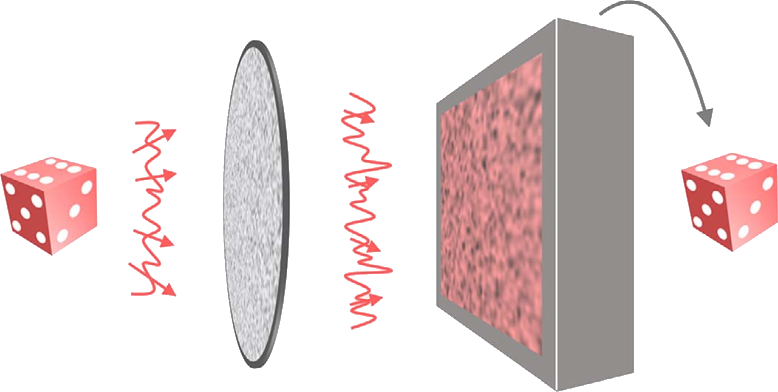## The speckle-correlation transmission matrix[K. Lee and Y. Park, Nat. Commun, 7 (2016)]

[Y. Baek, K. Lee and Y. Park, Phys. Rev. Appl., 7 (2016)]

[L. Gong, Q. Zhao, H. Zhang, X.-Y. Hu, K. Huang, J.-M. Yang and Y.-M. Li, Light Sci. Appl., 8 (2019)]

Measuring the optical phase is a ubiquitous challenge in optics. Through a linear scattering medium, one can always link the output optical field to the input one using the transmission matrix. However, one still has to measure the phase of the complex output field. In [K. Lee and Y. Park, Nat. Commun, 7 (2016)] the authors introduce a technique to reconstruct a complex optical field using a thin diffuser. Once the matrix is calibrated, only an intensity measurement is required to reconstruct the amplitude and the phase of the complex optical field.

Let's consider a scattering sample. Using various techniques we partially covered in the previous tutorials, one can measure its transmission matrix $$\mathbf{T}$$. If we call $$u_p$$, $$p\in [1...M]$$ the input basis elements, the input field can be expressed

$${E_{in}} = \sum_p^M c_p u_p$$

The resulting output complex field after propagation through the medium reads

$$E_{out} = \sum_p^M c_p t_p$$

with $$t_p$$ is the $$p^\text{th}$$ column of the transmission matrix $$\mathbf{T}$$, i.e. the complex output field corresponding to the injection of the $$p^\text{th}$$ element $$u_p$$ of the input basis.

The problem is the following, knowing the transmission matrix $$\mathbf{T}$$, how can we recover the complex input fields $$E_{in}$$, represented by its coefficients $$c_p$$, by only measuring the output intensity $$I_{out}=E_{out}E_{out}^*$$ ?

In [K. Lee and Y. Park, Nat. Commun, 7 (2016)], the authors introduced an operator they call the speckle-correlation transmission matrix $$\mathbf{Z}$$ represented by its coefficients

$$\mathbf{Z}_{pq}=\frac{1}{\left\langle\lvert t_p\rvert^2\right\rangle_r\left\langle\lvert t_q\rvert^2\right\rangle_r} \left[ \left\langle t_p^* t_q E_{out}^* E_{out} \right\rangle_r-\left\langle t_p^* t_q\right\rangle_r\left\langle E_{out}^* E_{out}\right\rangle_r \right]$$

$$\left\langle .\right\rangle_r$$ represents the spatial averaging.

Each element of this matrix takes the form of a 4-field correlator. What is very remarkable is that unlike standardly studied correlators, it does not involve two intensities, but one intensity ($$E_{out}^* E_{out}$$) and two different fields ($$t_p^*$$ and $$t_q$$). Using some assumptions about the transmission matrix (Gaussian distribution), and the speckle patterns, we obtain

$$\mathbf{Z}_{pq}\approx c_p c_q^*$$

It follows that this matrix has a rank equal to one and its unique singular vector is the incident field in the input basis representation $$c_p$$, $$p\in [1...M]$$.

Experimentally, after calibration of the transmission matrix $$\mathbf{T}$$, one can measure the output intensity pattern $$I_{out}$$ for a given unknown complex field and calculate the elements of the matrix $$\mathbf{Z}$$. A singular value decomposition is performed and the singular vector corresponding to the highest singular value is taken as a measure of the input field.

This approach, introduced in [K. Lee and Y. Park, Nat. Commun, 7 (2016)], was then successfully applied for lens-less holographic microscopy [Y. Baek, K. Lee and Y. Park, Phys. Rev. Appl., 7 (2016)] by the same group. It was then used for sending optical signals through a thin diffuser using a multiplexing scheme based on the orbital angular momentum in [L. Gong, Q. Zhao, H. Zhang, X.-Y. Hu, K. Huang, J.-M. Yang and Y.-M. Li, Light Sci. Appl., 8 (2019)].

Created by sebastien.popoff on 13/05/2019